﻿ 互联网环境下企业关键消费者选取比例优化策略研究 Optimization of Key Opinion Consumer Selection Strategy for Enterprise in the Internet Environment

Management Science and Engineering
Vol. 08  No. 04 ( 2019 ), Article ID: 33652 , 8 pages
10.12677/MSE.2019.84045

Optimization of Key Opinion Consumer Selection Strategy for Enterprise in the Internet Environment

Bilu Shen, Changjun Wang*

Glorious Sun School of Business and Management, Donghua University, Shanghai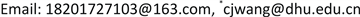Received: Dec. 4th, 2019; accepted: Dec. 19th, 2019; published: Dec. 26th, 2019ABSTRACT

With the rapid development of “people + content” as the core of social marketing, the product promotion for consumer network is one of the key issues that enterprises focus on. Scholars’ existing research on it is mainly based on the diffusion model to achieve the maximum marketing effect. However, it lacks a demand generation model based on consumer network, which can be used for marketing optimization. Thus, a nonlinear optimization model for Key Opinion Consumer (KOC) selection of proportion decision is constructed, using the Local Mean Field (LMF) method. Finally, simulations on different consumer purchase threshold, and combination of unit product profit and marketing cost are performed. The results show as following: 1) the smaller the ratio of unit marketing cost to product profit is, the larger the KOC ratio is, the larger the company’s market share will be and the more revenue it will generate; 2) when the unit marketing cost is higher than the product profit, the decision maker should strictly control the number of KOC for appropriate marketing, and then expand the market share.

Keywords:Key Opinion Consumer (KOC), Social Marketing, Local Mean Field (LMF), Consumer Network1. 引言

KOC能高效率地将公域流量转化为私域流量，最终推动消费者购买。因此，企业为快速推广新产品，实现自身利润最大化，精准选取KOC至关重要。而KOC极强的带货能力本质源于借助平台信息传播及互动。企业选取KOC需要付出一定成本，如何平衡营销成本与获益情况呢？Domingos和Richardson  最早提出了信息传播与影响最大化问题存在密切联系，在企业中的应用是“广告”、“口碑传播”  、“病毒式营销” 。其中，独立级联(Independent Cascade, IC)模型和线性阈值(Linear Threshold, LT)模型是最受学术研究者广泛关注的信息传播模型 。随后，基于这两种模型，学者们展开了大量关于病毒式营销的相关研究。如Kempe  证明了IC和LT模型下的病毒式营销决策问题为NP-hard，并设计了相应的贪婪算法。蔡国永和裴广战  基于经典LT模型，考虑邻居节点的间接影响力，提出LT + 影响力模型和LT + DAG算法。蔡淑琴等  考虑了消费者网络异质性，消费者的感知阈值与其退出传播的概率等因素，基于LT模型与企业价值共创理论，构建了传播响应模型(diffusion and responding model)，然而，其未考虑实际情景，结合企业收益最大化的目标进行决策研究。王长军等  利用LT模型描述了消费者在网络中的相互影响过程，探究渠道整合与产品体验性对O2O市场的影响。Singh  指出顾客互动在社交网络上的传播对信息传播和影响力方面具有关键作用，针对KOC的选取，提出了离散粒子群优化算法，并实验证明了其在LT、IC模型中的有效性。简言之，IC、LT等模型可考虑消费者网络具体结构以及消费者个体间的微观细节，但其为仿真模型，仅能通过启发式算法对精准营销进行决策，无法实现企业KOC营销的优化。

2. 模型构建

2.1. 消费者网络的构建

${\tau }_{\left(k\right)}^{0}=\frac{{k}^{-\lambda }}{{\sum }_{k=1}^{\infty }{k}^{-\lambda }}$ , $\left(k\in {N}^{+},\lambda \in \left(2,3\right)\right)$ (1)

${\tau }_{\left(k\right)}^{1}=\frac{{k}^{1-\lambda }}{{\sum }_{k=1}^{\infty }{k}^{1-\lambda }}$ , $\left(k\in {N}^{+},\lambda \in \left(2,3\right)\right)$ (2)

2.2. KOC选取优化决策模型构建

$\theta =\beta \lambda +\alpha \left(1-\lambda \right)$ , $\left(0\le \theta \le 1\right)$ (3)

$\left\{\begin{array}{l}{Y}_{i}=1,\text{\hspace{0.17em}}\text{\hspace{0.17em}}\text{\hspace{0.17em}}\text{\hspace{0.17em}}\text{ }\text{ }\underset{j}{\sum }{Y}_{j}\ge m,\text{\hspace{0.17em}}j=1,\cdots ,\text{deg}\left(i\right)\\ {Y}_{i}={\varphi }_{i},\text{\hspace{0.17em}}\text{\hspace{0.17em}}\text{\hspace{0.17em}}\underset{j}{\sum }{Y}_{j} (4)

$1-{Y}_{i}=\left(1-{\varphi }_{i}\right)\cdot 1\left[\underset{j=1}{\overset{\text{deg}\left(i\right)}{\sum }}{Y}_{j} (5)

$1-X=\left(1-{\varphi }_{r}\right)\cdot 1\left[\underset{j=1}{\overset{\text{deg}\left(r\right)}{\sum }}{Y}_{j} (6)

$\begin{array}{c}1-E\left[Y\right]=\left(1-\theta \right)E\left[1\left[\underset{j=1}{\overset{\text{deg}\left(i\right)}{\sum }}{Y}_{j} (7)

$\begin{array}{c}1-E\left[X\right]=\left(1-\theta \right)E\left[1\left[\underset{j=1}{\overset{\mathrm{deg}\left(r\right)}{\sum }}{Y}_{j} (8)

$E\left[X\right]$$E\left[Y\right]$ 分别指代根节点vr和非根节点vi在m-threshold下被激活的期望。特别地，对于LMF而言， $E\left[X\right]$ 表示产品信息扩散结束后整个消费者市场最终购买比例。

$z=\underset{\lambda ,E\left[X\right],E\left[Y\right]}{\mathrm{max}}\left\{E\left[X\right]×p×N-\lambda ×N×c|\left(3\right),\left(7\right),\left(8\right),0\le E\left[X\right],E\left[Y\right],\lambda \le 1\right\}$ (9)

3. 模拟仿真与结果分析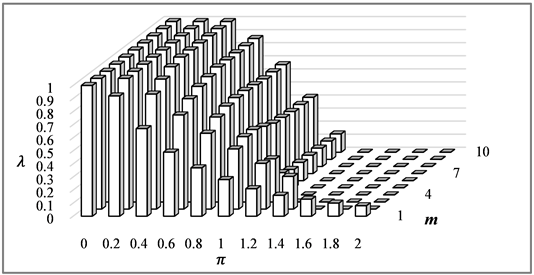Figure 1. Enterprise marketing decision $\lambda$ in different combination of $\pi$ and m

( $W=\left(E\left[X\right]-\theta \right)/\theta$ )。考虑到 $\mu$ 是企业进行营销决策后，消费者互动传播产品信息前市场期初的购买期望，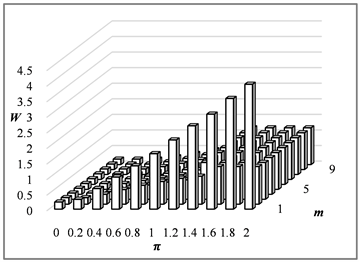(a)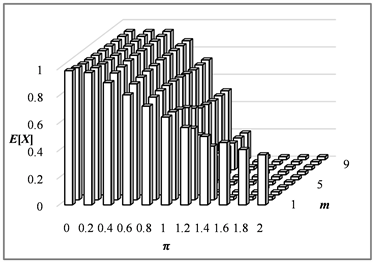(b)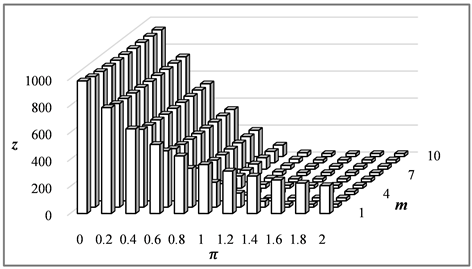(c)

Figure 2. Marketing results of W, E[X] and z in different combination of $\pi$ and m. (a) Promotion capacity W; (b) Market share E[X]; (c) Enterprise revenue z

1) 产品推广成本对企业的营销决策影响较大，营销力度与其成反比。然而，当单位产品推广成本高于产品利润时，企业并非不进行营销。特别是在消费者购买阈值较低的情况下，适度选取一定比例的KOC可大幅推广产品信息，拉动消费者购买需求，从而获得收益。

2) 产品推广成本与产品利润比越小时，企业加大营销力度有利于扩大其市场份额和增加收益。该结论可以由相关企业做法得以印证：荣耀9X不再单向投放广告，而是借助快手平台，利用其KOC与用户互动，以较低的成本引发巨大流量。即选取合理比例的KOC开拓市场，从而获得乐观的收益。

4. 结论与展望

Optimization of Key Opinion Consumer Selection Strategy for Enterprise in the Internet Environment[J]. 管理科学与工程, 2019, 08(04): 368-375. https://doi.org/10.12677/MSE.2019.84045

1. 1. Stephan, S., Jennifer, S. and Jimmy, H. (2014) Social Ecommerce. O’Reilly Media, ‎Sebastopol‎, CA.

2. 2. Peng, L., Cui, G., Chung, Y. and Li, C.Y. (2019) A Multi-Facet Item Response Theory Approach to Improve Customer Satisfaction Using Online Product Ratings. Journal of the Academy of Marketing Science, 47, 960-976.
https://doi.org/10.1007/s11747-019-00662-w

3. 3. Wang, J.K., Wang, X.H. and Li, Y. (2019) A Discrete Electronic Word-of-Mouth Propagation Model and Its Application in Online Social Networks. Physica A—Statistical Mechanics and Its Applications, 527, Article ID: 121172.
https://doi.org/10.1016/j.physa.2019.121172

4. 4. He, J., Han, M., Ji, S., Du, T.Y. and Li, Z. (2019) Spreading Social Influence with Both Positive and Negative Opinions in Online Networks. Big Data Mining and Analytics, 2, 30-47.
https://doi.org/10.26599/BDMA.2018.9020034

5. 5. Domingos, P. and Richardson, M. (2001) Mining the Network Value of Customers. Proceedings of the Seventh ACM SIGKDD International Conference on Knowledge Discovery and Data Mining, San Francisco, CA, 26-29 August 2001, 57-66.
https://doi.org/10.1145/502512.502525

6. 6. Kiss, C. and Bichler, M. (2009) Identification of Influencers—Measuring Influence in Customer Networks. Decision Support Systems, 46, 233-253.
https://doi.org/10.1016/j.dss.2008.06.007

7. 7. Babaei, M., Mirzasoleiman, B., Jalili, M. and Ali Safari, M. (2013) Revenue Maximization in Social Networks through Discounting. Social Network Analysis & Mining, 3, 1249-1262.
https://doi.org/10.1007/s13278-012-0085-5

8. 8. Jalili, M. and Perc, M. (2017) Information Cascades in Complex Networks. Journal of Complex Networks, 5, 665-693.
https://doi.org/10.1093/comnet/cnx019

9. 9. Kempe, D., Kleinberg, J. and Tardos, É. (2003) Maximizing the Spread of Influence through a Social Network. In: Getoor, L., Senator, T., et al., Eds., Proceeding of the Ninth ACM SIGKDD International Conference on Knowledge Discovery and Data Mining, ACM Press, Seattle, WA, 137-146.

10. 10. 蔡国永, 裴广战. 基于LT+模型的社交网络影响力最大化研究[J]. 计算机科学, 2016, 43(9): 99-102.

11. 11. 蔡淑琴, 袁乾, 周鹏. 企业响应下负面口碑线性阈值传播模型研究[J]. 系统工程学报, 2017, 32(2): 145-155.

12. 12. 王长军, 王葛格, 邓欣蕾. 考虑产品体验性和渠道整合的实物型产品O2O演化仿真研究[J]. 预测, 2018, 37(3): 75-80.

13. 13. Singh, S.S., Kumar, A., Singh, K. and Biswas, B. (2019) LAPSO-IM: A Learning-Based Influence Maximization Approach for Social Networks. Applied Soft Computing, 82, Article ID: 105554.
https://doi.org/10.1016/j.asoc.2019.105554

14. 14. Aldous, D. and Steele, J.M. (2004) The Objective Method-Probabilistic Combinatorial Optimization and Local Weak Convergence. Probability on Discrete Structures, 110, 29-35.
https://doi.org/10.1007/978-3-662-09444-0_1

15. 15. Barabasi, A.L. and Albert, R. (1999) Emergence of Scaling in Random Networks. Science, 286, 509-512.
https://doi.org/10.1126/science.286.5439.509

16. 16. Lelarge, M. and Bolot, J. (2008) A Local Mean Field Analysis of Security Investments in Networks. In: Joan, F. and Richard, Y.Y., Eds., Proceedings of the third International Workshop on Economics of Networked Systems, ACM Press, Seattle, WA, 25-30.
https://doi.org/10.1145/1403027.1403034

17. 17. Li, Y., Zhao, B.Q. and Lui, J.C.S. (2012) On Modeling Product Advertisement in Large-Scale Online Social Networks. IEEE/ACM Transactions on Networking, 20, 1412-1425.
https://doi.org/10.1109/TNET.2011.2178078

NOTES

*通讯作者。